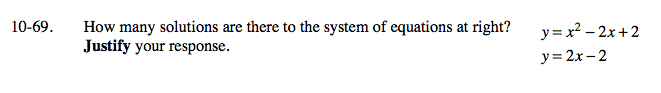### Home > A2C > Chapter 10 > Lesson 10.2.1 > Problem10-69

10-69.

How many solutions are there to the system of equations below? Justify your response. Homework Help ✎ y = x2 − 2x + 2 y = 2x − 2Substitute for y and solve:

(x − 2) = x2 − 2x + 2

Once you find x, remember to substitute it back intoone of the original equations to find the corresponding y-value.